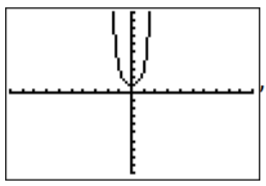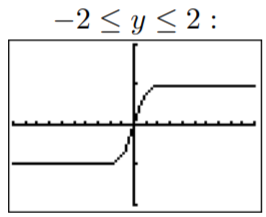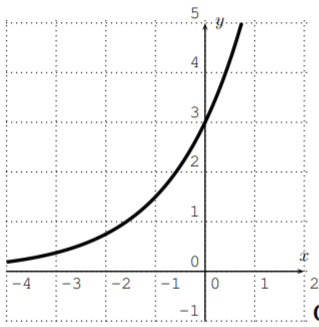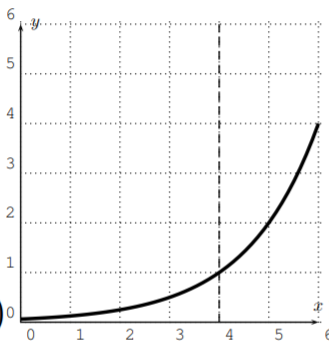# 13.3: Exercises

$$\newcommand{\vecs}{\overset { \rightharpoonup} {\mathbf{#1}} }$$ $$\newcommand{\vecd}{\overset{-\!-\!\rightharpoonup}{\vphantom{a}\smash {#1}}}$$$$\newcommand{\id}{\mathrm{id}}$$ $$\newcommand{\Span}{\mathrm{span}}$$ $$\newcommand{\kernel}{\mathrm{null}\,}$$ $$\newcommand{\range}{\mathrm{range}\,}$$ $$\newcommand{\RealPart}{\mathrm{Re}}$$ $$\newcommand{\ImaginaryPart}{\mathrm{Im}}$$ $$\newcommand{\Argument}{\mathrm{Arg}}$$ $$\newcommand{\norm}{\| #1 \|}$$ $$\newcommand{\inner}{\langle #1, #2 \rangle}$$ $$\newcommand{\Span}{\mathrm{span}}$$ $$\newcommand{\id}{\mathrm{id}}$$ $$\newcommand{\Span}{\mathrm{span}}$$ $$\newcommand{\kernel}{\mathrm{null}\,}$$ $$\newcommand{\range}{\mathrm{range}\,}$$ $$\newcommand{\RealPart}{\mathrm{Re}}$$ $$\newcommand{\ImaginaryPart}{\mathrm{Im}}$$ $$\newcommand{\Argument}{\mathrm{Arg}}$$ $$\newcommand{\norm}{\| #1 \|}$$ $$\newcommand{\inner}{\langle #1, #2 \rangle}$$ $$\newcommand{\Span}{\mathrm{span}}$$$$\newcommand{\AA}{\unicode[.8,0]{x212B}}$$

## Exercise $$\PageIndex{1}$$

Graph the following functions with the calculator.

1. $$y=5^x$$
2. $$y=1.01^x$$
3. $$y=\left (\dfrac 1 3 \right )^x$$
4. $$y=0.97^x$$
5. $$y=3^{-x}$$
6. $$y=\left (\dfrac 1 3 \right )^{-x}$$
7. $$y=e^{x^2}$$
8. $$y=0.01^x$$
9. $$y=1^x$$
10. $$y=e^{x}+1$$
11. $$y=\dfrac{e^x-e^{-x}}{2}$$
12. $$y=\dfrac{e^x-e^{-x}}{e^x+e^{-x}}$$

The last two functions are known as the hyperbolic sine, $$\sinh(x)=\dfrac{e^x-e^{-x}}{2}$$, and the hyperbolic tangent, $$\tanh(x)=\dfrac{e^x-e^{-x}}{e^x+e^{-x}}$$. Recall that the hyperbolic cosine $$\cosh(x)=\dfrac{e^x+e^{-x}}{2}$$ was already graphed in example hyperbolic-cosine.

1.2.3.4.5. same as c) since $$y=\left(\dfrac{1}{3}\right)^{x}=3^{-x}$$
6.7.8.9.10.11.12.## Exercise $$\PageIndex{2}$$

Graph the given function. Describe how the graph is obtained by a transformation from the graph of an exponential function $$y=b^x$$ (for appropriate base $$b$$).

1. $$y=0.1\cdot 4^x$$
2. $$y=3\cdot 2^x$$
3. $$y=(-1)\cdot 2^x$$
4. $$y=0.006\cdot 2^x$$
5. $$y=e^{-x}$$
6. $$y=e^{-x}+1$$
7. $$y=(\dfrac 1 2)^{x}+3$$
8. $$y=2^{x-4}$$
9. $$y=2^{x+1}-6$$
1. $$y=4^{x}$$ is compressed towards the x-axis by the factor $$0.1$$2. $$y = 2^x$$ stretched away from $$x$$-axis3. $$y = 2^x$$ reflected about the $$x$$-axis4. $$y = 2^x$$ compressed towards the $$x$$-axis5. $$y = e^x$$ reflected about the $$y$$-axis6. $$y = e^x$$ reflected about the $$y$$-axis and shifted up by $$1$$7. $$y=\left(\dfrac{1}{2}\right)^{x}$$ shifted up by $$3$$8. $$y = 2^x$$ shifted to the right by $$4$$9. $$y = 2^x$$ shifted to the left by $$1$$ and down by $$6$$## Exercise $$\PageIndex{3}$$

Use the definition of the logarithm to write the given equation as an equivalent logarithmic equation.

1. $$4^2=16$$
2. $$2^{8}=256$$
3. $$e^x=7$$
4. $$10^{-1}=0.1$$
5. $$3^x=12$$
6. $$5^{7\cdot x}=12$$
7. $$3^{2a+1}=44$$
8. $$\left(\dfrac{1}{2}\right)^{\frac{x}{h}}=30$$
1. $$\log _{4}(16)=2$$
2. $$\log _{2}(256)=8$$
3. $$\ln (7)=x$$
4. $$\log (0.1)=-1$$
5. $$\log _{3}(12)=x$$
6. $$\log _{5}(12)=7 x$$
7. $$\log _{3}(44)=2 a+1$$
8. $$\log _{\frac{1}{2}}(30)=\dfrac{x}{h}$$

## Exercise $$\PageIndex{4}$$

Evaluate the following expressions without using a calculator.

1. $$\log_7(49)$$
2. $$\log_3(81)$$
3. $$\log_{2}(64)$$
4. $$\log_{50}(2,500)$$
5. $$\log_2(0.25)$$
6. $$\log(1,000)$$
7. $$\ln(e^4)$$
8. $$\log_{13}(13)$$
9. $$\log(0.1)$$
10. $$\log_6 \left (\dfrac 1 {36} \right)$$
11. $$\ln(1)$$
12. $$\log_{\frac 1 2}(8)$$
1. $$2$$
2. $$4$$
3. $$6$$
4. $$2$$
5. $$−2$$
6. $$3$$
7. $$4$$
8. $$1$$
9. $$−1$$
10. $$−2$$
11. $$0$$
12. $$−3$$

## Exercise $$\PageIndex{5}$$

Using a calculator, approximate the following expressions to the nearest thousandth.

1. $$\log_3(50)$$
2. $$\log_3(12)$$
3. $$\log_{17}(0.44)$$
4. $$\log_{0.34}(200)$$
1. $$3.561$$
2. $$2.262$$
3. $$−0.290$$
4. $$−4.911$$

## Exercise $$\PageIndex{6}$$

State the domain of the function $$f$$ and sketch its graph.

1. $$f(x)=\log(x)$$
2. $$f(x)=\log(x+7)$$
3. $$f(x)=\ln(x+5)-1$$
4. $$f(x)=\ln(3x-6)$$
5. $$f(x)=2\cdot \log(x+4)$$
6. $$f(x)=-4\cdot\log(x+2)$$
7. $$f(x)=\log_{3}(x-5)$$
8. $$f(x)=-\ln(x-1)+3$$
9. $$f(x)=\log_{0.4}(x)$$
10. $$f(x)=\log_{3}(-5x)-2$$
11. $$f(x)=\log|x|$$
12. $$f(x)=\log|x+2|$$
1.2.3.4.5.6.7.8.9.10.11.12.This page titled 13.3: Exercises is shared under a CC BY-NC-SA 4.0 license and was authored, remixed, and/or curated by Thomas Tradler and Holly Carley (New York City College of Technology at CUNY Academic Works) via source content that was edited to the style and standards of the LibreTexts platform; a detailed edit history is available upon request.